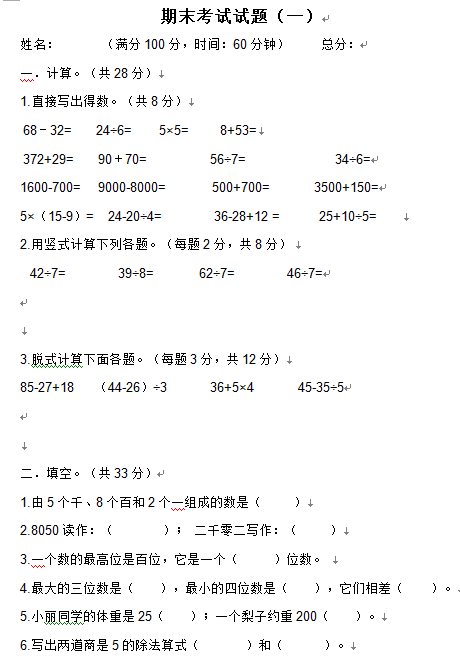ceomax主题已启用，当前站点还没有验证正版主题授权，暂不可使用 前往授权激活 获取正版授权
【DOC文档4页】小学二年级数学下册期末考试试题(一)A4电子版资料_可直接打印_会员免费下载 - 悟思学_领悟_思考_学习

【DOC文档4页】小学二年级数学下册期末考试试题(一)A4电子版资料_可直接打印_会员免费下载

266

1.直接写出得数。（共8分）
68－32=      24÷6=       5×5=        8+53=
372+29=      90＋70=            56÷7=              34÷6=

1600-700=    9000-8000=       500+700=       3500+150=

5×（15-9）=    24-20÷4=          36-28+12 =      25+10÷5=

【DOC文档4页】小学二年级数学下册期末考试试题(一)A4电子版资料_可直接打印_会员免费下载相关资料206 5244 53842

• 0 +

访问总数

• 0 +

会员总数

• 0 +

文档总数

• 0 +

今日发布

• 0 +

本周发布

• 0 +

运行天数